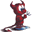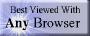music# expressions

## summary

This subchapter looks at expressions.

## free computer programming text book project

If you like the idea of this project,### stub section

This subchapter is a stub section. It will be filled in with instructional material later. For now it serves the purpose of a place holder for the order of instruction.

Professors are invited to give feedback on both the proposed contents and the propsed order of this text book. Send commentary to Milo, PO Box 1361, Tustin, California, 92781, USA.

# expressions

This subchapter looks at expressions.

### assembly language instructions

• EMOD Extended Multiply and Integerize; DEC VAX; performs accurate range reduction of math function arguments, the floating point multiplier extension operand (second operand) is concatenated with the floating point multiplier (first operand) to gain eight additional low order fraction bits, the multiplicand operand (third operand) is multiplied by the extended multiplier operand, after multiplication the integer portion (fourth operand) is extracted and a 32 bit (EMODF) or 64 bit (EMODD) floating point number is formed from the fractional part of the product by truncating extra bits, the multiplication is such that the result is equivalent to the exact product truncated (before normalization) to a fraction field of 32 bits in floating or 64 bits in double (fifth operand); clears or sets flags
• POLY Polynomial Evaluation; DEC VAX; performs fast calculation of math functions, for degree times (second operand) evaluate a function using Horner’s method, where d=degree (second operand), x=argument (first operand), and result = C + x*(C + x*(C + … x*C[d])), float result stored in D0 register, double float result stored in D0:D1 register pair, the table address operand (third operand) points to a table of polynomial coefficients ordered from highest order term of the polynomial through lower order coefficients stored at increasing addresses, the data type of the coefficients must be the same as the data type of the argument operand (first operand), the unsigned word degree operand (second operand) specifies the highest numbered coefficient to participate in the evaluation (POLYF polynomial evaluation floating, POLYD polynomial evaluation double float); D0 through D4 registers modified by POLYF, D0 through D5 registers modified by POLYD; sets or clears flags
• TBLS Table Lookup and Interpolate (Signed, Rounded); Motorola 68300; signed lookup and interpolation of independent variable X from a compressed linear data table or between two register-based table entries of linear representations of dependent variable Y as a function of X; ENTRY(n) + {(ENTRY(n+1) - ENTRY(n)) * Dx[7:0]} / 256 into Dx; table version: data register low word contains the independent variable X, 8-bit integer part and 8-bit fractional part with assumed radix point located between bits 7 and 8, source effective address points to beginning of table in memory, integer part scaled to data size (byte, word, or longword) and used as offset from beginning of table, selected table entry (a linear representation of dependent variable Y) is subtracted from the next consecutive table entry, then multiplied by the interpolation fraction, then divided by 256, then added to the first table entry, and then stored in the data register; register version: data register low byte contains the independent variable X 8-bit fractional part with assumed radix point located between bits 7 and 8, two data registers contain the byte, word, or longword table entries (a linear representation of dependent variable Y), first data register-based table entry is subtracted from the second data register-based table entry, then multiplied by the interpolation fraction, then divided by 256, then added to the first table entry, and then stored in the destination (X) data register, the register interpolation mode may be used with several table lookup and interpolations to model multidimentional functions; rounding is selected by the ‘R’ instruction field, for a rounding adjustment of -1, 0, or +1; interpolation resolution is limited to 1/256th the distance between consecutrive table entries, X should be considered an integer in the range 0 ≤ X ≤ 65535; sets or clears flags
• TBLSN Table Lookup and Interpolate (Signed, Not Rounded); Motorola 68300; signed lookup and interpolation of independent variable X from a compressed linear data table or between two register-based table entries of linear representations of dependent variable Y as a function of X; ENTRY(n) * 256 + (ENTRY(n+1) - ENTRY(n)) * Dx[7:0] into Dx; table version: data register low word contains the independent variable X, 8-bit integer part and 8-bit fractional part with assumed radix point located between bits 7 and 8, source effective address points to beginning of table in memory, integer part scaled to data size (byte, word, or longword) and used as offset from beginning of table, selected table entry (a linear representation of dependent variable Y) multiplied by 256, then added to the value determined by (selected table entry subtracted from the next consecutive table entry, then multiplied by the interpolation fraction), and then stored in the data register; register version: data register low byte contains the independent variable X 8-bit fractional part with assumed radix point located between bits 7 and 8, two data registers contain the byte, word, or longword table entries (a linear representation of dependent variable Y), first data register-based table entry is multiplied by 256, then added to the value determined by (first data register-based table entry subtracted from the second data register-based table entry, then multiplied by the interpolation fraction), and then stored in the destination (X) data register, the register interpolation mode may be used with several table lookup and interpolations to model multidimentional functions; the result is an 8-, 16-, or 24-bit integer and eight-bit fraction; interpolation resolution is limited to 1/256th the distance between consecutrive table entries, X should be considered an integer in the range 0 ≤ X ≤ 65535; sets or clears flags
• TBLU Table Lookup and Interpolate (Unsigned, Rounded); Motorola 68300; unsigned lookup and interpolation of independent variable X from a compressed linear data table or between two register-based table entries of linear representations of dependent variable Y as a function of X; ENTRY(n) + {(ENTRY(n+1) - ENTRY(n)) * Dx[7:0]} / 256 into Dx; table version: data register low word contains the independent variable X, 8-bit integer part and 8-bit fractional part with assumed radix point located between bits 7 and 8, source effective address points to beginning of table in memory, integer part scaled to data size (byte, word, or longword) and used as offset from beginning of table, selected table entry (a linear representation of dependent variable Y) is subtracted from the next consecutive table entry, then multiplied by the interpolation fraction, then divided by 256, then added to the first table entry, and then stored in the data register; register version: data register low byte contains the independent variable X 8-bit fractional part with assumed radix point located between bits 7 and 8, two data registers contain the byte, word, or longword table entries (a linear representation of dependent variable Y), first data register-based table entry is subtracted from the second data register-based table entry, then multiplied by the interpolation fraction, then divided by 256, then added to the first table entry, and then stored in the destination (X) data register, the register interpolation mode may be used with several table lookup and interpolations to model multidimentional functions; rounding is selected by the ‘R’ instruction field, for a rounding adjustment of 0 or +1; the result is an 8-, 16-, or 24-bit integer and eight-bit fraction; interpolation resolution is limited to 1/256th the distance between consecutrive table entries, X should be considered an integer in the range 0 ≤ X ≤ 65535; sets or clears flags
• TBLUN Table Lookup and Interpolate (Unsigned, Not Rounded); Motorola 68300; unsigned lookup and interpolation of independent variable X from a compressed linear data table or between two register-based table entries of linear representations of dependent variable Y as a function of X; ENTRY(n) * 256 + (ENTRY(n+1) - ENTRY(n)) * Dx[7:0] into Dx; table version: data register low word contains the independent variable X, 8-bit integer part and 8-bit fractional part with assumed radix point located between bits 7 and 8, source effective address points to beginning of table in memory, integer part scaled to data size (byte, word, or longword) and used as offset from beginning of table, selected table entry (a linear representation of dependent variable Y) multiplied by 256, then added to the value determined by (selected table entry subtracted from the next consecutive table entry, then multiplied by the interpolation fraction), and then stored in the data register; register version: data register low byte contains the independent variable X 8-bit fractional part with assumed radix point located between bits 7 and 8, two data registers contain the byte, word, or longword table entries (a linear representation of dependent variable Y), first data register-based table entry is multiplied by 256, then added to the value determined by (first data register-based table entry subtracted from the second data register-based table entry, then multiplied by the interpolation fraction), and then stored in the destination (X) data register, the register interpolation mode may be used with several table lookup and interpolations to model multidimentional functions; the result is an 8-, 16-, or 24-bit integer and eight-bit fraction; interpolation resolution is limited to 1/256th the distance between consecutrive table entries, X should be considered an integer in the range 0 ≤ X ≤ 65535; sets or clears flags
• CRC Calculate Cyclic Redundancy Check; DEC VAX; performs a cyclic redundancy check (CRC) on the string designated by the length and memory address, using the designated table holding the CRC polynomial and the designated initial CRC; uses R0 through R4 and leaves answer in R0; sets or clears flags

# free music player coding example

Coding example: I am making heavily documented and explained open source code for a method to play music for free — almost any song, no subscription fees, no download costs, no advertisements, all completely legal. This is done by building a front-end to YouTube (which checks the copyright permissions for you).

View music player in action: www.musicinpublic.com/.

Create your own copy from the original source code/ (presented for learning programming).

## view text bookHTML file

Because I no longer have the computer and software to make PDFs, the book is available as an HTML file, which you can convert into a PDF.

 Tweets by @osdata

## free computer programming text book project

Building a free downloadable text book on computer programming for university, college, community college, and high school classes in computer programming.

If you like the idea of this project,

send donations to:
Milo
PO Box 1361
Tustin, California 92781

Supporting the entire project:

If you have a business or organization that can support the entire cost of this project, please contact Pr Ntr Kmt (my church)

Some or all of the material on this web page appears in theThis web site handcrafted on Macintoshcomputers using Tom Bender’s Tex-Edit Plusand served using FreeBSD.†UNIX used as a generic term unless specifically used as a trademark (such as in the phrase “UNIX certified”). UNIX is a registered trademark in the United States and other countries, licensed exclusively through X/Open Company Ltd.

Names and logos of various OSs are trademarks of their respective owners.